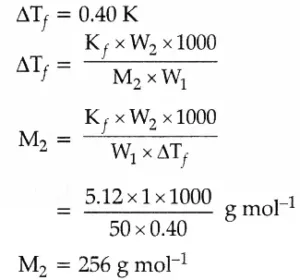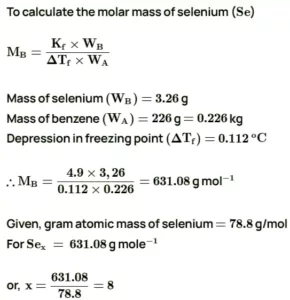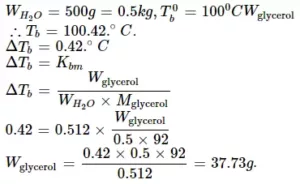# Solutions Chemistry Class 12 Important Questions with AnswersSolutions Chemistry Class 12 Important Questions with Answers

Q1. a) Calculate the molarity of a sulphuric acid solution in which the mole fraction of water is 0.85.
b) The graphical representation of vapour pressure of two-component system as a function of composition is given alongside.
i) Are the A – B interactions weaker, stronger or of the same magnitude as A–A and B–B
ii) Name the type of deviation shown by this system from Raoult’s law.
iii) Predict the sign of ∆mixH for this system.
iv) Predict the sign of ∆mixV for this system.
v) Give an example of such a system.
vi) What type of azeotrope will this system form, if possible?Ans 1 (a). X1 = 0.85
X2 = 1 – 0.85 = 0.15
1 Molal solution is the number of moles dissolved per thousand grams of solvent
Number of moles of solvent, n1 = 1000/ 18 = 55.55 mol
X2 = n2 /(n1 + n2) or 0.15 = n2 / (55.55 + n2)
Therefore n2 = 9.8
(b). i) Stronger
ii) Negative deviation
iii) Negative
iv) Negative
v) 20% acetone and 80% chloroform by mass
vi) Maximum boiling azeotrope

Que 2. a) State Raoult’s Law for a solution containing volatile components. How does Raoult’s law become a special case of Henry’s Law?
b) 1.00 g of a nonelectrolyte solute dissolved in 50 g of benzene lowered the freezing point of benzene by 0.40 K. Find the molar mass of the solute. (Kf for benzene = 5.12 K kg mol–1).
Ans 2. (a). For a solution of volatile liquids, Raoult’s law states that the partial vapour pressure of each component of the solution is directly proportional to its mole fraction present in the solution,
i.e., pA α xA
pA = p0AxA
According to Henry’s Law, the partial pressure of a gas in the vapour phase (p) is directly proportional to the mole fraction(x) of the gas in the solution.
i.e., p = KHx on comparing it with Raoult’s Law it can be seen that partial pressure of the volatile component or gas is directly proportional to its mole fraction in solution
i.e; p α x only the proportionality constant K differs from p0. Thus, it becomes a special case of Henry’s law in which K = p0.
(b). Kf = 5.12 K k/mol
W1 = 50 g
W2 = 1.0 g
ΔTf = 0.4 KSolutions Chemistry Class 12 Important Questions with Answers

Que 3. The molar freezing point depression constant for benzene is 4.90K kg mol–1. Selenium exists as polymer SeX. When 3.26 gm of Se is dissolved in 226 gm of benzene, the observed freezing point is 0.112 0C lower than for pure benzene. Decide the molecular formula of Selenium. (At. wt. of selenium is 78.8 g mol1).
Ans 3.Que 4. Determine the amount of CaCl2 (i = 2.47) dissolved in 2.5 litres of water such that its osmotic pressure is 0.75 atm at 27C.
Ans 4. We know that
π = i n/V RT
⇒π = i w/MV iRT
⇒ w = πMV /  iRT     …………………..(1)
Now we have given below values:
π =  0.75 atm
V = 2.5L
i = 2.47
T = (27+273) K = 300K
Here,
R = 0.0821 L atm k-1 mol-1
M = 1 × 40 + 2 × 35.5
= 111 g/mol
Now putting the value in equation 1:
w =  0.75 × 111 × 2.5/2.47 × 0.0821 × 300
=3.42g
Hence, the required amount of CaCl2 is 3.42 g.

Solutions Chemistry Class 12 Important Questions with Answers

Que 5. A solution of glycerol (C3H8O3) in water was prepared by dissolving some glycerol in 500g of water. This solution has a boiling point of 100.42 0C while pure water boils at 100 0C. What mass of glycerol was dissolved to make the solution? (Kb of water = 0.512 Kkg/mol).
Ans 5.Que 6. a) Why an increase in temperature observed on mixing chloroform and acetone?
b) Why does sodium chloride solution freeze at a lower temperature than water?
Ans 6. (a). The bonds between chloroform molecules and molecules of acetone are dipole-dipole interactions, but on mixing, the chloroform and acetone molecules start forming hydrogen bonds which are stronger bonds resulting in the release of energy. This gives rise to an increase in temperature.
(b). The freezing point of a liquid depresses on the addition of a non-volatile solute, and therefore, a solution of sodium chloride freezes at a temperature lower than the freezing point of water. On the other hand, there is an elevation of the boiling point on the addition of a non-volatile solute and consequently, the boiling point of sodium chloride solution is more than that of water.

Que 7. Non-ideal solution exhibit either positive or negative deviations from Raoult’s law. What are these deviations and why are they caused? Explain with one example for each type.
Ans 7. When the vapour pressure of a solution is either higher or lower than that predicted by Raoult’s law, then the solution exhibits deviation from Raoult’s law. These deviations are caused when solute-solvent molecular interactions A–B are either weak or stronger than solvent – solvent A–B or solute–solute B – B molecular interactions.
Positive deviations: When A – B molecular interactions are weaker than A – A and B – B molecular interaction. For example, a mixture of ethanol and acetone.
Negative deviations: When A – B molecular interaction are stronger than A – A and B – B molecular interaction. For example, a mixture of chloroform and acetone.

Solutions Chemistry Class 12 Important Questions with Answers

Que 8. Suggest the most important type of intermolecular interaction in the following pairs:
i) N-hexane and n-octane
ii) Methanol and acetone
Ans 8. i) Dispersion or London forces as both are non-polar.
ii) Dipole-dipole interactions as both are polar molecules.

Que 9. How the measurement of osmotic pressure is more widely used for determining molar masses of macromolecules than the elevation in boiling point or depression in freezing point of their solutions?
Ans 9. The osmotic pressure method has the advantage over elevation in boiling point or depression in freezing point for determining molar masses of macromolecules because
(i) Osmotic pressure is measured at room temperature and the molarity of a solution is used instead of morality.
(ii) Compared to other colligative properties, its magnitude is large even for very dilute solutions.

Que 10. State Henry’s law. What is the significance of KH?
Ans 10. According to this Law “, the partial pressure of the gas in the vapour phase (p) is directly proportional to the mole fraction of the gas (x ) in the solution”.

Que 11. What is the value of van’t Hoff factor for a dilute solution of
(i) K2SO4 in Water                    (ii) Acetic acid in Benzene.
Ans 11. (i) 3 (ii) 1/2

Solutions Chemistry Class 12 Important Questions with Answers

Que 12. Why is the osmotic pressure of 1M KCl higher than 1M urea solution?
Ans 12. This is because KCl dissociates to give K + and Cl– ions while urea being a molecular solid does not dissociate into ions in the solution.

Que 13. What are minimum boiling azeotropes? Give one example.
Ans 13. Minimum boiling azeotropes are those which boil at a lower temperature than the boiling point of each component in a pure state. e.g., 95.5 % ethyl alcohol and 4.5 % water by mass.

Que 14. What do you understand by the term that Kf for water is 1.86 Kkg/mol?
Ans 14. It means that the freezing point of water is lowered by1.86 K when 1 mol of non-volatile solute is dissolved in 1kg of water.

Que 15. How does the molarity of a solution change with temperature?
Ans 15. Molarity decreases with an increase in temperature as the volume of the solution increase with the increase in temperature.

Que 16. Under what condition do non-ideal solutions show negative deviation?
Ans 16. When the new forces of interaction between the components are stronger than those in the pure components, then non-ideal solutions show negative deviation.

Solutions Chemistry Class 12 Important Questions with Answers